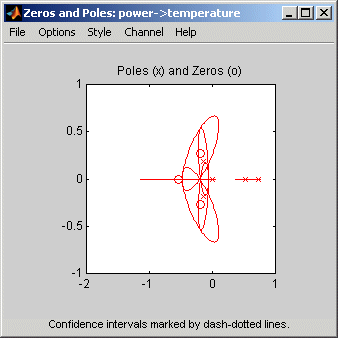## Pole and Zero Plots

### Supported Models

You can create pole-zero plots of linear identified models. To study the poles and zeros of the noise component of an input-output model or a time series model, use `noise2meas` to first extract the noise model as an independent input-output model, whose inputs are the noise channels of the original model.

For examples of creating pole-zero plots, see Model Poles and Zeros Using the System Identification App and Plot Poles and Zeros at the Command Line.

### What Does a Pole-Zero Plot Show?

The following figure shows a sample pole-zero plot of the model with confidence intervals. `x` indicate poles and `o` indicate zeros.The general equation of a linear dynamic system is given by:

`$y\left(t\right)=G\left(z\right)u\left(t\right)+v\left(t\right)$`

In this equation, G is an operator that takes the input to the output and captures the system dynamics, and v is the additive noise term.

The poles of a linear system are the roots of the denominator of the transfer function G. The poles have a direct influence on the dynamic properties of the system. The zeros are the roots of the numerator of G. If you estimated a noise model H in addition to the dynamic model G, you can also view the poles and zeros of the noise model.

Zeros and the poles are equivalent ways of describing the coefficients of a linear difference equation, such as the ARX model. Poles are associated with the output side of the difference equation, and zeros are associated with the input side of the equation. The number of poles is equal to the number of sampling intervals between the most-delayed and least-delayed output. The number of zeros is equal to the number of sampling intervals between the most-delayed and least-delayed input. For example, there two poles and one zero in the following ARX model:

### Displaying the Confidence Interval

You can display a confidence interval for each pole and zero on the plot. To learn how to show or hide confidence interval, see Model Poles and Zeros Using the System Identification App and Plot Poles and Zeros at the Command Line.

The confidence interval corresponds to the range of pole or zero values with a specific probability of being the actual pole or zero of the system. The toolbox uses the estimated uncertainty in the model parameters to calculate confidence intervals and assumes the estimates have a Gaussian distribution.

For example, for a 95% confidence interval, the region around the nominal pole or zero value represents the range of values that have a 95% probability of being the true system pole or zero value. You can specify the confidence interval as a probability (between 0 and 1) or as the number of standard deviations of a Gaussian distribution. For example, a probability of 0.99 (99%) corresponds to 2.58 standard deviations.

You can use pole-zero plots to evaluate whether it might be useful to reduce model order. When confidence intervals for a pole-zero pair overlap, this overlap indicates a possible pole-zero cancellation. For more information, see Reducing Model Order Using Pole-Zero Plots.

## Related Topics

##### Support평가판 신청